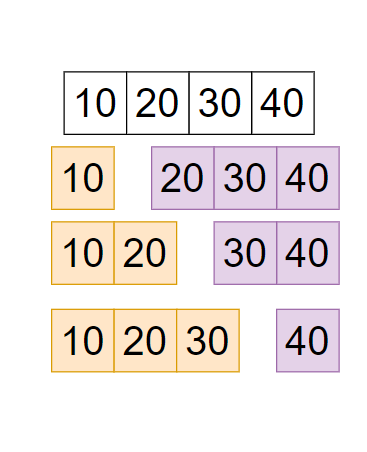# Allocate Books

Posted: 12 Jan, 2021
Difficulty: Moderate

## PROBLEM STATEMENT

#### ‘arr = { 10, 20, 30, 40 }’.#### But you have to return a minimum of this so return ‘min(90,70,60) =60’.

##### Note:
``````1. Do not print anything, just return the maximum number of pages that are assigned to a student is minimum.
2. If it is not possible to assign the ‘n’ books to ‘m’ students then return ‘-1’.
``````
##### Input format:
``````The first line of input contains an integer ‘T’ denoting the number of test cases.
The next ‘2*T’ lines represent the ‘T’ test cases.

The first line of each test case contains two space-separated integers ‘n’ denoting the number of books and ‘m’ denotes the number of students.

The second line of each test case contains ‘n’ space-separated integers denoting the number of pages in each of ‘n’ books.
``````
##### Output Format
``````For each test case, return the minimum number of pages.
``````
##### Constraints:
``````1 <= T <= 50
2 <= M <= N <= 10^3
1 <= A[i] <= 10^9
Sum of all A[i] do not more than 10^9.

Where ‘T’ is the total number of test cases, ‘N’ denotes the number of books and ‘M’ denotes the number of students. ‘A[i]’ denotes an element at position ‘i’ in the sequence.

Time limit: 1 second
``````Approach 1

The basic idea is that, try each and every possible value that can be answered. It can be from ‘1’ to the sum of ‘arr’.

• Find the sum of all the elements of ‘arr’ in a variable ‘sum’.
• Iterate a loop ‘i’ from ‘1’ to ‘sum’(inclusive).
• Check for every ‘i’, if it is possible to divide the at least ‘i’ pages to every student.
• If possible then return ‘i’ because we are iterating from minimum then it is the minimum answer.
• Else check for ‘i+1’.
• If the number of ‘n<m’ then return ‘-1’, because the number of students is more than the number of books.

#### How to check - if it is possible to divide at least ‘mid’ number of pages in each student

Iterate a loop ‘i’, if sum of some contiguous books pages is less than or equals to mid then assign this sou to  a single student  and check for remain student and at the end if is possible then can say it is possible that ‘mid’ number of pages is possible to assign ‘m’ student.